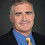## Thursday, August 14, 2014

### Favorite Theorems: Limited Independence

When can limited randomness act as well as true random bits?
Polylogarithmic independence fools AC0 circuits by Mark Braverman (JACM 2010)
To explain this result consider choosing uniformly from among the following four strings:
000 110 101 011
If we look at any two of the bits, say the first and third, all four possibilities 00 10 11 01 occur. The sequence is thus 2-wise independent. We can get 2-wise independence using only two random bits to choose one of the four strings. In general one can get k-wise independent in n-bit strings using O(k2 log n) random bits.

Braverman shows that any polylogarithmic independent distribution fools polynomial-size constant-depth circuits (AC0), or more precisely for a size s depth d circuit C, for k=(log (m/ε))O(d2), the probability that C will output 1 with uniformly-random inputs will differ at most ε from the probability C outputs 1 from inputs chosen from a k-wise random distribution. Braverman's result followed after Bazzi and Razborov proved a similar result for depth-2 circuits (CNF formulas).

Another nice result along these lines: Ilias Diakonikolas, Parikshit Gopalan, Ragesh Jaiswal, Rocco Servedio and Emanuele Viola show that Bounded Independence Fools Halfspaces. A half-space is just a weighted threshold function (is the sum of wixi at most some given θ). Diakonikolas et al. show that one can fool halfspaces with k=O(ε-2log2(1/ε)), in particular for constant ε, k is a constant independent of the number of variables.

1.Only k\lg n random bits are needed for k-wise independent n-bit strings, see for example Vadhan's monograph (http://people.seas.harvard.edu/~salil/pseudorandomness/ , section 3.5.5).

2.Bazzi has provided some information on the genesis of his paper, see http://emanueleviola.wordpress.com/2014/08/02/the-sandwich-revolution-behind-the-paper/

3.I haven't read the paper, but based on your description of the result, is an informal description that "any polylogarithmic independent distribution fools polynomial-size constant-depth circuits" justified? It sounds like the result establishes the existence of a deceptive polylogarithmic-independent distribution.

4.Adding emphasis to the previous comment for clarity... Based on your description of the result, is an informal description that "*any* polylogarithmic independent distribution fools polynomial-size constant-depth circuits" justified? It sounds like the result establishes the *existence* of a deceptive polylogarithmic-independent distribution.

1.Braverman does prove that every polylogarithmic independent distribution will fool poly-size constant depth circuits. I recommend reading Braverman's well-written paper, particularly the introduction, for more details.